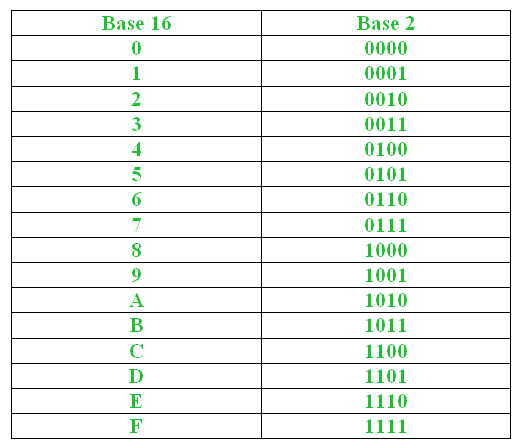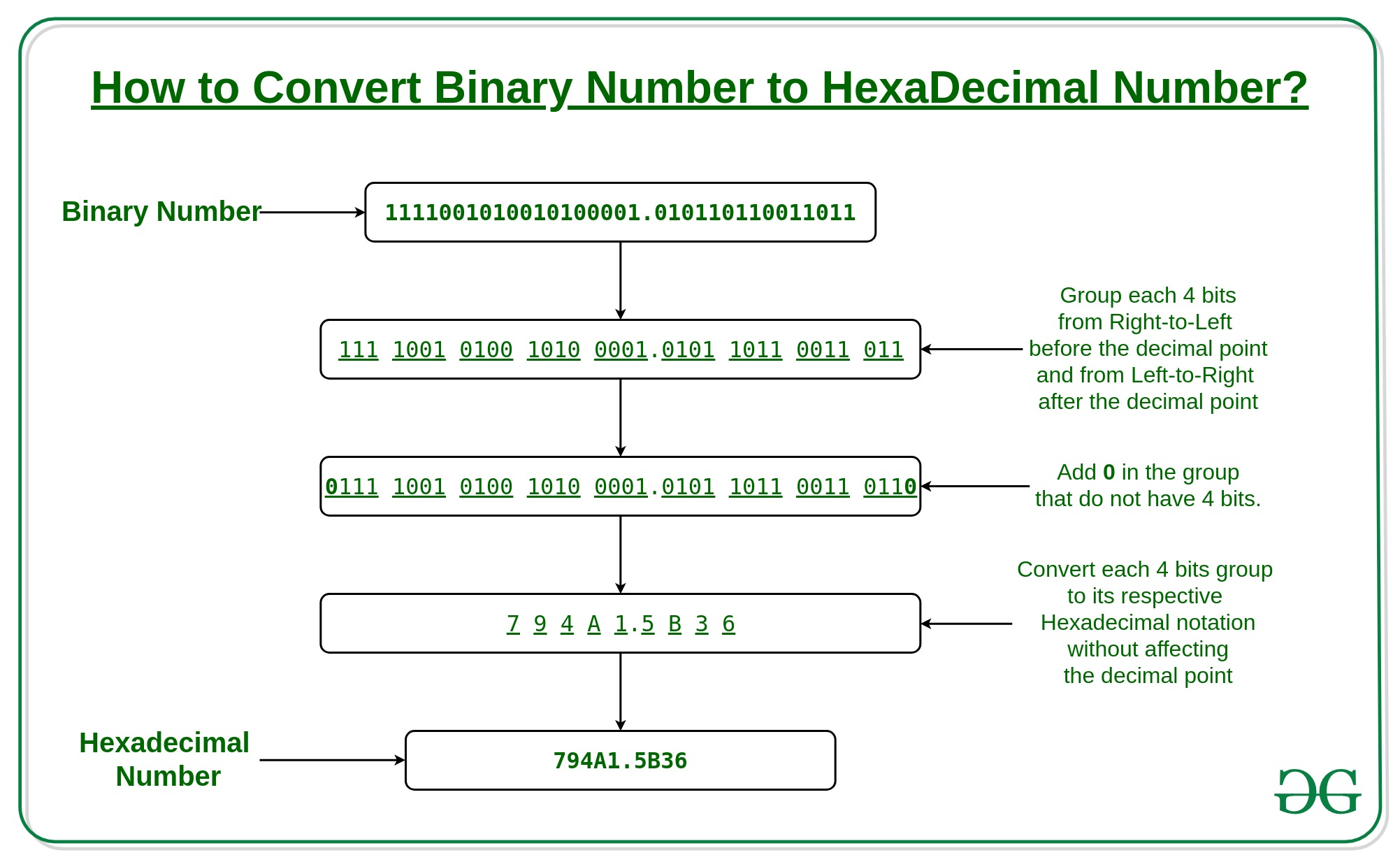Related Articles

# Convert a binary number to hexadecimal number

• Difficulty Level : Easy
• Last Updated : 17 Nov, 2020

Given a Binary Number, the task is to convert the given binary number to its equivalent hexadecimal number. The input could be very large and may not fit even into an unsigned long long int.

Examples:

```Input: 110001110
Output: 18E

Input: 1111001010010100001.010110110011011
Output: 794A1.5B36

```

Approach 1:
Binary Number: A binary number is a number expressed in the base-2 binary numeral system, which uses only two symbols: which are 0 (zero) and 1 (one).

4HexaDecimal Number: A hexadecimal number is a positional numeral system with a radix, or base, of 16 and uses sixteen distinct symbols: which are 0, 1, 2, 3, 4, 5, 6, 7, 8, 9, A, B, C, D, E, and F.We all know that, 24 = 16 1
In other words, single digit in base 16 can be represented using 4 digits in base 2.To convert Binary number to HexaDecimal, the below steps are taken:

1. Group the given Binary Number into groups of 4 bits, each group taken individually from the left and right of the decimal point.
2. Get length of substring to the left and right of the decimal point(‘.’) as left_len and right_len.
3. If left_len is not a multiple of 4, i.e., grouping into exact group of 4 bits is not possible, then add minimum number of 0’s in the beginning to make length of left substring a multiple of 4.
4. Similarly, If right_len is not a multiple of 4, then add minimum number of 0’s in the end to make length of right substring a multiple of 4.
5. Now, from the left, extract each group (substrings of length 4) one by one and add its corresponding Hexadecimal code to the result.
6. If in between a decimal(‘.’) is ecountered then add it to the result.

Below is the implementation of the above approach:

## C++

 `// C++ implementation to``// convert a binary number to hexadecimal number` `#include ``using` `namespace` `std;` `// Function to create map between binary``// number and its equivalent hexadecimal``void` `createMap(unordered_map *um)``{``    ``(*um)[``"0000"``] = ``'0'``;``    ``(*um)[``"0001"``] = ``'1'``;``    ``(*um)[``"0010"``] = ``'2'``;``    ``(*um)[``"0011"``] = ``'3'``;``    ``(*um)[``"0100"``] = ``'4'``;``    ``(*um)[``"0101"``] = ``'5'``;``    ``(*um)[``"0110"``] = ``'6'``;``    ``(*um)[``"0111"``] = ``'7'``;``    ``(*um)[``"1000"``] = ``'8'``;``    ``(*um)[``"1001"``] = ``'9'``;``    ``(*um)[``"1010"``] = ``'A'``;``    ``(*um)[``"1011"``] = ``'B'``;``    ``(*um)[``"1100"``] = ``'C'``;``    ``(*um)[``"1101"``] = ``'D'``;``    ``(*um)[``"1110"``] = ``'E'``;``    ``(*um)[``"1111"``] = ``'F'``;``}` `// function to find hexadecimal``// equivalent of binary``string convertBinToHex(string bin)``{``    ``int` `l = bin.size();``    ``int` `t = bin.find_first_of(``'.'``);``    ` `    ``// length of string before '.'``    ``int` `len_left = t != -1 ? t : l;``    ` `    ``// add min 0's in the beginning to make``    ``// left substring length divisible by 4``    ``for` `(``int` `i = 1; i <= (4 - len_left % 4) % 4; i++)``        ``bin = ``'0'` `+ bin;``    ` `    ``// if decimal point exists   ``    ``if` `(t != -1)   ``    ``{``        ``// length of string after '.'``        ``int` `len_right = l - len_left - 1;``        ` `        ``// add min 0's in the end to make right``        ``// substring length divisible by 4``        ``for` `(``int` `i = 1; i <= (4 - len_right % 4) % 4; i++)``            ``bin = bin + ``'0'``;``    ``}``    ` `    ``// create map between binary and its``    ``// equivalent hex code``    ``unordered_map bin_hex_map;``    ``createMap(&bin_hex_map);``    ` `    ``int` `i = 0;``    ``string hex = ``""``;``    ` `    ``while` `(1)``    ``{``        ``// one by one extract from left, substring``        ``// of size 4 and add its hex code``        ``hex += bin_hex_map[bin.substr(i, 4)];``        ``i += 4;``        ``if` `(i == bin.size())``            ``break``;``            ` `        ``// if '.' is encountered add it``        ``// to result``        ``if` `(bin.at(i) == ``'.'``)   ``        ``{``            ``hex += ``'.'``;``            ``i++;``        ``}``    ``}``    ` `    ``// required hexadecimal number``    ``return` `hex;   ``}` `// Driver program to test above``int` `main()``{``    ``string bin = ``"1111001010010100001.010110110011011"``;``    ``cout << ``"Hexadecimal number = "``         ``<< convertBinToHex(bin);``    ``return` `0;    ``}`

## Java

 `// Java implementation to convert a``// binary number to hexadecimal number``import` `java.io.*;``import` `java.util.*;` `class` `GFG{` `// Function to create map between binary``// number and its equivalent hexadecimal``static` `void` `createMap(Map um)``{``    ``um.put(``"0000"``, ``'0'``);``    ``um.put(``"0001"``, ``'1'``);``    ``um.put(``"0010"``, ``'2'``);``    ``um.put(``"0011"``, ``'3'``);``    ``um.put(``"0100"``, ``'4'``);``    ``um.put(``"0101"``, ``'5'``);``    ``um.put(``"0110"``, ``'6'``);``    ``um.put(``"0111"``, ``'7'``);``    ``um.put(``"1000"``, ``'8'``);``    ``um.put(``"1001"``, ``'9'``);``    ``um.put(``"1010"``, ``'A'``);``    ``um.put(``"1011"``, ``'B'``);``    ``um.put(``"1100"``, ``'C'``);``    ``um.put(``"1101"``, ``'D'``);``    ``um.put(``"1110"``, ``'E'``);``    ``um.put(``"1111"``, ``'F'``);``}` `// Function to find hexadecimal``// equivalent of binary``static` `String convertBinToHex(String bin)``{``    ``int` `l = bin.length();``    ``int` `t = bin.indexOf(``'.'``);` `    ``// Length of string before '.'``    ``int` `len_left = t != -``1` `? t : l;` `    ``// Add min 0's in the beginning to make``    ``// left substring length divisible by 4``    ``for``(``int` `i = ``1``;``            ``i <= (``4` `- len_left % ``4``) % ``4``;``            ``i++)``        ``bin = ``'0'` `+ bin;` `    ``// If decimal point exists``    ``if` `(t != -``1``)``    ``{``        ` `        ``// Length of string after '.'``        ``int` `len_right = l - len_left - ``1``;` `        ``// Add min 0's in the end to make right``        ``// substring length divisible by 4``        ``for``(``int` `i = ``1``;``                ``i <= (``4` `- len_right % ``4``) % ``4``;``                ``i++)``            ``bin = bin + ``'0'``;``    ``}` `    ``// Create map between binary and its``    ``// equivalent hex code``    ``Map bin_hex_map = ``new` `HashMap();``    ``createMap(bin_hex_map);` `    ``int` `i = ``0``;``    ``String hex = ``""``;` `    ``while` `(``true``)``    ``{``        ` `        ``// One by one extract from left, substring``        ``// of size 4 and add its hex code``        ``hex += bin_hex_map.get(``            ``bin.substring(i, i + ``4``));``        ``i += ``4``;``        ` `        ``if` `(i == bin.length())``            ``break``;` `        ``// If '.' is encountered add it``        ``// to result``        ``if` `(bin.charAt(i) == ``'.'``)``        ``{``            ``hex += ``'.'``;``            ``i++;``        ``}``    ``}` `    ``// Required hexadecimal number``    ``return` `hex;``}` `// Driver code``public` `static` `void` `main(String[] args)``{``    ``String bin = ``"1111001010010100001.010110110011011"``;``    ` `    ``System.out.print(``"Hexadecimal number = "` `+``                      ``convertBinToHex(bin));``}``}` `// This code is contributed by jithin`

Output:

```Hexadecimal number = 794A1.5B36

```

Time Complexity: O(n), where n is the length of the string.
Approach 2: Another approach to convert Binary Number to Hexadecimal number is to first convert the binary number to decimal number and then convert the obtained decimal number to equivalent hexadecimal number.
Practice Questions:
(1) Convert the binary number 111000 to hexa-decimal.
(2) Convert the binary number 100100001 to hexa-decimal.
(3) Convert the binary number 1001001111 to hexa-decimal.
(4) What is the binary equivalent of hexa-decimal number A7C5.
(5) What is the binary equivalent of hexa-decimal number 2A.FF.

```Answers:
(1) 38
(2) 121
(3) 24F
(4) 1010011111000101
(5) 101010.11111111

```

This article is contributed by Ayush Jauhari. If you like GeeksforGeeks and would like to contribute, you can also write an article using contribute.geeksforgeeks.org or mail your article to contribute@geeksforgeeks.org. See your article appearing on the GeeksforGeeks main page and help other Geeks.